Reach Us+44 1704 335730
Ekf- Based Sensorless Direct Torque Control Of Permanent Magnet Synchronous Motor: Comparison Between Two Different Selection Tables | OMICS International
Global Journal of Technology and Optimization
All submissions of the EM system will be redirected to Online Manuscript Submission System. Authors are requested to submit articles directly to Online Manuscript Submission System of respective journal.

# Ekf- Based Sensorless Direct Torque Control Of Permanent Magnet Synchronous Motor: Comparison Between Two Different Selection Tables

Habib Kraiem*, Mustapha Messaoudi, Lassaâd Sbita and Mohamed Naceur Abdelkrim

National Engineering School of Gabes (ENIG), Tunisia

*Corresponding Author:
Habib Kraiem
National Engineering School of Gabes (ENIG), Tunisia
E-mail: [email protected], [email protected]

Received date: April 2009; Revised date: June 2009; Accepted date: November 2009

Visit for more related articles at Global Journal of Technology and Optimization

#### Abstract

In this paper a sensorless direct torque control (DTC) scheme of permanent magnet synchronous motor (PMSM) is proposed. To improve the performance of the classical DTC a modified control scheme based on twelve sectors instead of six is presented and a comparison between the two methods is carried out. The high performance of the DTC is related to the accuracy of the flux estimation witch is affected by parameter variation especially the stator resistance due changes in temperature or frequency. Therefore, it is adequate to compensate this parameter variation using an online adaptation of the control scheme by the estimated stator resistance using the Extended Kalman Filter (EKF). The EKF is designed to estimate the rotor speed and stator flux and resistance. Estimated parameters are used for the closed loop speed sensorless control operation of the PMSM. It has been demonstrated that the EKF estimation and sensorless DTC perform quite well in spite of the parameters and load variations that handled by the system. The simulation results are presented to validate the effectiveness of the overall control scheme.

#### Keywords

DTC, Synchronous machine, EKF, Sensor-less, PM

#### Introduction

In high-performance servo applications a rapid and accurate torque control is desired, preferably without the use of a motion-state sensor . The use of PMSMs combined with the DTC scheme offers many opportunities to achieve this goal.

The appellation synchronous motor is derived from the fact that the rotor and the rotating field of the stator rotate together at the same speed. The rotor tends to align itself with the rotating field produced by the stator. The stator has often a three phase winding. The rotor magnetization is caused by the permanent magnets in the rotor. These motors types are called permanent magnet synchronous motors (PMSMs).

PMSMs are widely used in high performance drives such as industrial robots and machine tools thanks to their known advantages as: low inertia, high efficiency, high power density, high reliability and free maintenance.

More than two decades ago, DTC was introduced by Takahashi for induction motors as an alternative to the field oriented control (FOC) technique , . This drive strategy can also be applied to PMSM. The idea of combining the advantages of DTC and PMSMs into a highly dynamic drive appeared in the literature in the late 1990’s .

The DTC strategy directly controls the inverter states based on the errors between the reference and estimated values of torque and flux. It selects one of eight voltage vectors generated by a voltage source inverter to keep torque and flux within the limits of two hysteresis comparators. Compared with Rotor Field Oriented Control, DTC has many advantages such as less machine parameter dependence (only the stator resistance), simpler implementation and quicker dynamic torque response. There is no current controller needed in DTC, because it selects the voltage space vectors according to the errors of flux linkage and torque. The main drawback of the DTC is its relatively high torque and flux ripple. To improve the performance of the classical DTC, the stator flux locus is divided into twelve sectors instead of six so all six active states will be used in each sector.

In the classical DTC, the stator flux vector which is estimated as the integral of the stator voltage vector as described by (5). This estimation suffers from the well known problems of the pure integration and on the other hand the stator resistance variation effect and this occur especially at low speed operation range . Therefore we propose in this work to use the EKF to observe simultaneously the rotor speed and stator flux and resistance.

This observation does not depend on motor parameter except the stator resistance. A constant value of stator resistance is considered. However, in practice, this parameter changes due to variation of stator windings temperature. This fact introduces errors in the flux and the electromagnetic torque estimations and the drive may become unstable. So the compensation of the stator resistance variation effect becomes necessary  . In this paper, the variation of stator resistance is online estimated and compensated using the EKF.

Basic Synchronous Motor Model

The dynamic behavior of a synchronous machine is described by the following equations written in space vector notation and in the d-q referential , :

Stator Voltage Equations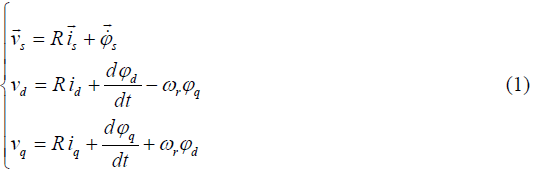Stator Flux EquationsElectromagnetic torque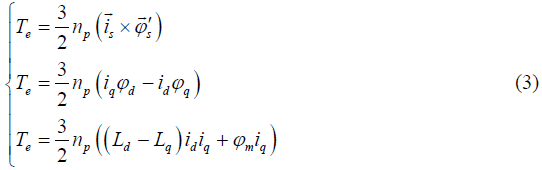Mechanical Equation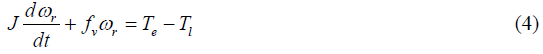Where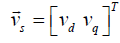is the stator voltage vector,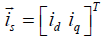is the stator current vector,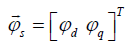is the stator flux vector,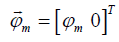is the permanent magnet flux linkage vector,

R is the stator resistance, Ld and Lq are the d and q axis self stator inductances, np the number of pole pairs, Te and Tl are the electromagnetic and load torques, J is the moment of inertia, ωr is the rotor speed and fv is the friction factor.

Direct Torque Control Strategy

DTC of PMSM is carried out by hysteresis control of motor stator flux and torque that directly select one of the six non-zero and two zero discrete voltage vectors of the inverter  Figure 1. The selection of the voltage vectors is made so as to restrict the motor stator flux and torque errors within the hysteresis band and obtain the fastest torque response . The selection of the voltage vectors in conventional DTC is based on error outputs produced by the torque and flux hysteresis controllers (τf and τT) and is provided in the form of a switching table as shown in Table (1).

Flux and Torque Estimation

In the conventional DTC scheme, the stator flux is gotten from (5), which is derived from (1) using only the measured stator voltages and currents .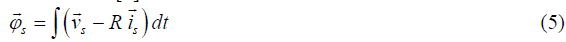This equation is the foundation for implementing the flux estimator. It may be implemented directly, or approximated by various methods to avoid integrator drift .

At average and high speed, the voltage drop term (R.is) can be neglected, so the stator flux expression becomes: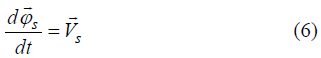Therefore the stator flux variation is null for a null voltage vector.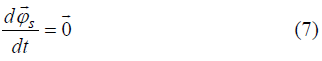DTC requires accurate knowledge of the amplitude and angular position for the flux controller: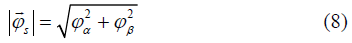The angular position of the stator flux vector must be known so that the DTC can chose between an appropriate set of vector depending on the flux position.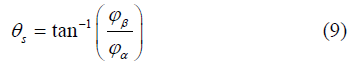The electromagnetic torque can be estimated by using the stator currents measurement and estimated flux as (10):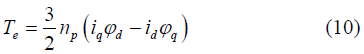Torque and flux hysterisis comparators

The electromagnetic torque value resulting from (10) is then compared with the reference electromagnetic torque, using the three level hysteresis comparator, represented in Figure 2. In this manner, the result may be increase, decrease or maintain the torque, depending on the comparator output , .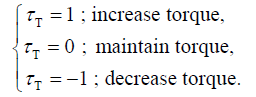In a similar way, the flux value will be compared with the reference flux, but using a two level hysteresis comparator, shown in Figure 3. The result will be used to increase or decrease the flux.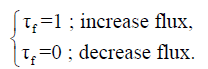An important factor in these operations is the hysteresis band of the two comparators. A too small value may have the effect of losing the control. The stator flux linkage may exceed the values required by the tolerance band. A narrow window will give better current and flux waveforms but will also increase the inverter switching frequency.

Voltage Source Inverter

The voltage source inverter can be modelled as shown in Figure 4, where Sa, Sb, Sc are the switching states (1 in case of switch-on, 0 in case of switch-off). Eight output voltage vectors V0 to V7 {000, 100, 110, 010, 011, 001, 101, 111} are obtained for different switch combinations. Hence, V0 and V7 are zero voltage vectors. The voltage vector of the three- phase voltage inverter is presented as follows: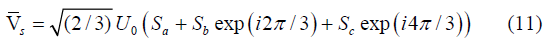The combination of the comparator states, (τT , τf ) and the position sector of the stator flux are used by the optimal switching selection table (1) to chose an appropriate voltage vector to be applied to the inverter .

Twelve sectors Table

In classical DTC, as shown by Figure 1, there are two states per sector that present a torque ambiguity. Therefore, they are not used. It can be seen in the first sector that the vectors V1 and V4, are not used in the classical DTC because they can increase or decrease the torque at the same sector depending on if the position is in its first 30 degrees or in its second ones. It seems a good idea that if the stator flux locus is divided into twelve sectors instead of just six, all six active states will be used per sector as shown in Table (2). Consequently, it is arisen the idea of the twelve sectors DTC. This novel stator flux locus is introduced in Figure 5 Notice how all six voltage vectors can be used in all twelve sectors. However, it has to be introduced the idea of small torque increase instead of torque increase, mainly due to the fact that the tangential voltage vector component is very small and consequently its torque variation will be small as well. Therefore, the hysteresis block should consist of four levels instead of two.

Extended Kalman Filter

The Kalman filter (KF) is a special kind of observer, which provides optimal filtering of noises in measurement and inside the system if the covariance matrices of these noises are known. The process and the measurement noises are both assumed to be Gaussian with a zero mean. The elements of their covariance matrices (Q and R) serve as design parameters for the convergence of the algorithm. For nonlinear problems, the KF is not strictly applicable since linearity plays an important role in its derivation and performance as an optimal filter. The EKF attempts to overcome this difficulty by using a linearized approximation where the linearization is performed about the current state estimate . The general diagram of the EKF is given by Figure 6.

Also the KF has the ability to produce estimates of states which are not measurable. This feature is particularly important for estimation problems associated with the PMSM as the rotor quantities are not directly accessible. If a simultaneous estimate of the machine parameter, let say stator resistance, is needed then it is defined as an auxiliary state variable. A new state vector containing the original states and the parameter is then established. In this case, the nonlinearity of the system increases. Therefore, the EKF is more convenient than the KF. Using the d-q coordinate system and separating the machine variables state vectors into their real and imaginary parts, the well-known PMSM model expressed in terms of the state variables is obtained from (1)-(4), and is given by :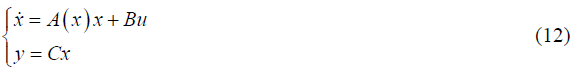Where x, u and y are respectively the state vector, the input vector and the output vector which are defined as fellow: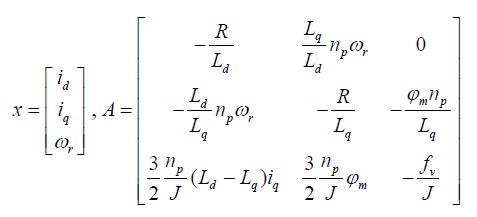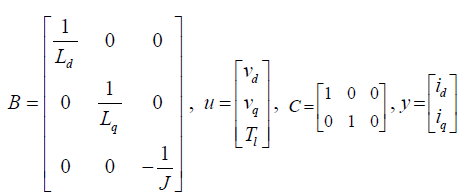The stator flux and the electromagnetic torque are not estimated directly using the EKF. Their estimations are done using respectively equations (2) and (3) and by exploiting the estimated stator current id and id.

Let us now see the recursive form of the EKF as in . Prediction: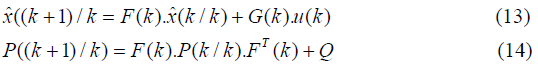Correction: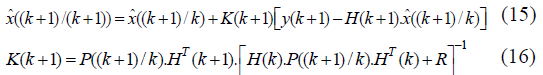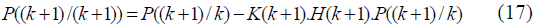Where the estimation covariance error is: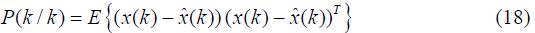K is the Kalman gain matrix. ((k+1)/k) denotes a prediction at time (k+1) based on data up to and including k. (14) and (17) forms the well-known Riccati equation.

Equation (12) defines a continuous model, but as estimation is to be implemented on a digital processor, the PMSM continuous model must be written in a discrete form. By applying the Euler formula a discrete time-varying non-linear model is obtained: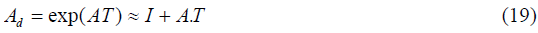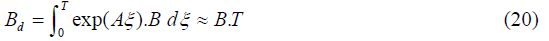The discrete time varying nonlinear stochastic model of the PMSM has the following form: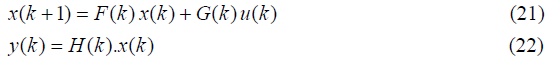Where x(k), u(k) and y(k) are defined as fellow: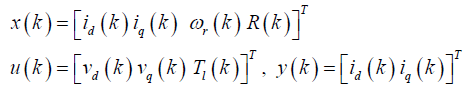The process and the measurement noise vectors are random variables and characterized by: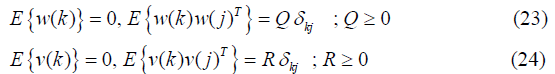The initial state x(0) is characterized by: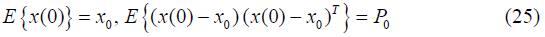Simulation Results

To verify the effectiveness of the proposed DTC sensorless PMSM drives as illustrated in Figure 7, a digital simulation based on Matlab- Simulink software package has been carried out. Motor parameters used in simulation are given in the appendix. The used sampling period for simulation is T = 10-5s.

Figure 8 to 11 show the rotor speed, the electromagnetic torque, the stator currents and flux responses to a triangular wave speed reference target. The flux reference is maintained at its nominal value of 0.3 Wb and a square wave load torque is applied. Figure 8 shows the actual and estimated responses of the proposed PMSM sensorless scheme. Torque control dynamic of the DTC is shown in Figure 9. It is noted that fast transient torque response is obtained owing to the fast dynamic of the hysteresis controller. It can be seen from Figure 11 that flux is independent from the torque variations and the estimated flux magnitude follows the reference value.

Figure 12 and 13 show respectively the simulation results of the estimation of the stator resistance and the effect of this parameter variation on the flux trajectory (R = 1.3*R at 0.7s). We can see that the position and the magnitude of stator flux are changed

Figure 13: The stator flux trajectory without compensation of the stator resistance variation.

The results in Figure 14 to 16 illustrate the rotor speed, the electromagnetic torque, and the stator current and flux simulation responses when a load torque step of 10 Nm at 0.5s is applied with compensation of the stator resistance variation. The target speed is changed from 0 rad/s to 157 rad/s at 0.1s. The result clearly shows that the estimated speed follows the actual one with a negligible error after load torque and stator resistance variation (Tl =10 N.m at 0.5s, R = 1.3*R at 0.7s). The trajectory of the estimated flux vector presented in Figure 17 shows that the compensation of the stator resistance variation is achieved; the magnitude and the position of stator flux are not affected when stator resistance is increased. The load torque variation did not affect the stator flux trajectory. The main drawback of the DTC is its relatively high torque and flux ripples as shown in Figure 15 and 17. In all simulation presented, it can be observed that EKF gives a good observation of the rotor speed, stator current, flux and resistance.

Figure 18, 19 and 20 presents a comparison between the classical DTC and the proposed twelve sectors DTC. It is clearly shown that, the novels DTC reduces the torque and flux ripples but there are not completely eliminated.

#### Conclusion

In this paper, modeling and simulation of Sensorless DTC of PMSM has been presented. EKF is designed and developed for use in closed loop control to estimate the rotor speed, stator flux and resistance. The filtering action of EKF improves the system performance in presence of stator resistance and load torque variations. The main advantages of this method are the high dynamics of torque control, simple control algorithm and short time of calculation. To overcome the major drawback of the DTC, witch is the torque and flux ripples, twelve sectors DTC scheme is used. It is clearly shown that the ripples are reduces but not completely eliminated.

#### Appendix

Three phases PMSM parameters: Rated output power 1500 Watt, Rated phase voltage 220/380V, φm=0.15Wb, R=1.4Ω, Ld= 0.0066H, Lq= 0.0058H, fv = 0.00038N.m.rad-1.s, J = 0.00176 kg.m2, np= 3.

#### References

Select your language of interest to view the total content in your interested language

### Article Usage

• Total views: 14932
• [From(publication date):
June-2010 - Nov 15, 2019]
• Breakdown by view type
• HTML page views : 11038Can't read the image? click here to refresh